## D. Kuziak, I. Peterin, I. G. Yero: On the monopolies of lexicographic product graphs: bounds and closed formulas, p. 355-366

### Abstract:

We consider a simple graph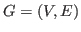without isolated vertices and of minimum degree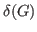. Let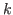be an integer number such that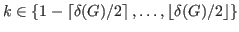. A vertex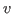of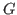is said to be-controlled by a set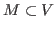, if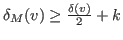where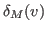represents the number of neighborshas in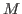and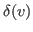the degree of. The setis called a-monopoly if it-controls every vertexof. The minimum cardinality of any-monopoly inis the-monopoly number of. In this article we study the-monopolies of the lexicographic product of graphs. Specifically we obtain several relationships between the-monopoly number of this product graph and the-monopoly numbers and/or order of its factors. Moreover, we bound (or compute the exact value) of the-monopoly number of several families of lexicographic product graphs.

Key Words: monopolies; lexicographic product graphs

2010 Mathematics Subject Classification: Primary 05C69,
Secondary 05C76